Mobile QR Code1. (School of Electronic and Electrical Engineering, Kyungpook National University, Daegu 702-201, Korea)

Multiple nanosheet tunneling field-effect transistor (MNSTFET), polycrystalline silicon (Poly-Si), grain boundary (GB)

## I. INTRODUCTION

Recently, as the size of the semiconductor devices has decreased, the short-channel effects (SCEs) has appeared in the metal-oxide-semiconductor field-effect transistors (MOSFETs) [1-4]. Also, a subthreshold swing (SS) of the MOSFET is limited to 60 mV/decade at a room temperature. However, because a tunneling field-effect transistor (TFET) operates by the band-to-band-tunneling (BTBT), it has the immunity to the SCEs and the SS under 60 mV/dec [5-8]. Also, the poly-Si can be applied to 3-dimensional vertical stacking, and is advantageous in terms of manufacturing process technology. In addition, it can replace the silicon-on-insulator (SOI) structure of the high fabrication cost. In this study, the multiple nanosheet structure based on the polycrystalline silicon (poly-Si) is used to improve the I$_{\mathrm{on}}$ of the TFET and to suppress the SCE. These multiple nanosheet tunneling field-effect transistors (MNSTFETs) based on the poly-Si can compensate for the low I$_{\mathrm{on}}$ of TFET. Because the poly-Si is adapted in these devices, it is important to analyze the grain boundary (GB)’s effects. [9,10]

In this study, we present the electrical performances of the MNSTFETs depending on the GB-locations. Also, we compare the electrical characteristics of the MNSTFETs and the MNSMOSFETs based on the poly-Si .

## II. DEVICE STRUCTURE AND SIMULATION METHOD

Fig. 1 shows the 3-dimensional view and the cross-sectional view of the MNSTFET based on the poly-Si . The poly-Si was used as a material for the nanosheet channels, and the gate length (L$_{\mathrm{g}}$), the nanosheet width (W$_{\mathrm{sh}}$), and the nanosheet total width (W$_{\mathrm{total}}$) are 16, 30, and 76 nm, respectively. The gate insulator (HfO$_{2}$/SiO$_{2}$) thickness (T$_{\mathrm{ox}}$) is 1.1/0.3 nm, and SiO$_{2}$ was used as the interfacial layer. The doping concentrations of the source, channel, and drain regions are 1 ${\times}$ 10$^{20}$ cm$^{-3}$ (p-type), 1 ${\times}$ 10$^{16}$ cm$^{-3}$ (n-type), and 1 ${\times}$ 10$^{19}$ cm$^{-3}$ (n-type), respectively. The parameters of the proposed device are summarized in Table 1. The I$_{\mathrm{on}}$ is defined as the drain current (I$_{\mathrm{DS}}$) when the gate voltage (V$_{\mathrm{GS}}$) is 1.0 V and the drain voltage (V$_{\mathrm{DS}}$) is 0.7 V. The I$_{\mathrm{off}}$ is defined as the I$_{\mathrm{DS}}$ when the V$_{\mathrm{GS}}$ = 0.0 V and the V$_{\mathrm{DS}}$ = 0.7 V. The V$_{\mathrm{T}}$ is defined as the gate voltage when the I$_{\mathrm{DS}}$ = 0.1 ${\mu}$A/${\mu}$m. The average of subthreshold swing (SS$_{\mathrm{ave}}$) is defined as the inverse of the average slope between two points where the I$_{\mathrm{DS}}$ is I$_{\mathrm{off}}$ and 0.1 ${\mu}$A/${\mu}$m.

Fig. 2(a) shows the I$_{\mathrm{DS}}$-V$_{\mathrm{GS}}$ simulation curves fitted by the experimental data in  to accurately implement the tunneling model. The trap distribution in the grain boundary (GB) of the poly-Si was extracted from experimental data from , as shown in Fig. 2(b). The GB has both the donor-like traps and the acceptor-like traps. This device was simulated by the Sentaurus technology computer-aided design (TCAD) tool. These simulations include the dynamic nonlocal BTBT model, the bandgap narrowing model, the Fermi-Dirac model, the Shockley-Read-Hall recombination model, the trap-assisted Auger recombination model, and the multivalley (MLDA) model to increase the accuracy of the simulation .

##### Fig. 1. The 3-dimensional view and the cross-sectional view of the MNSTFET based on the poly-Si.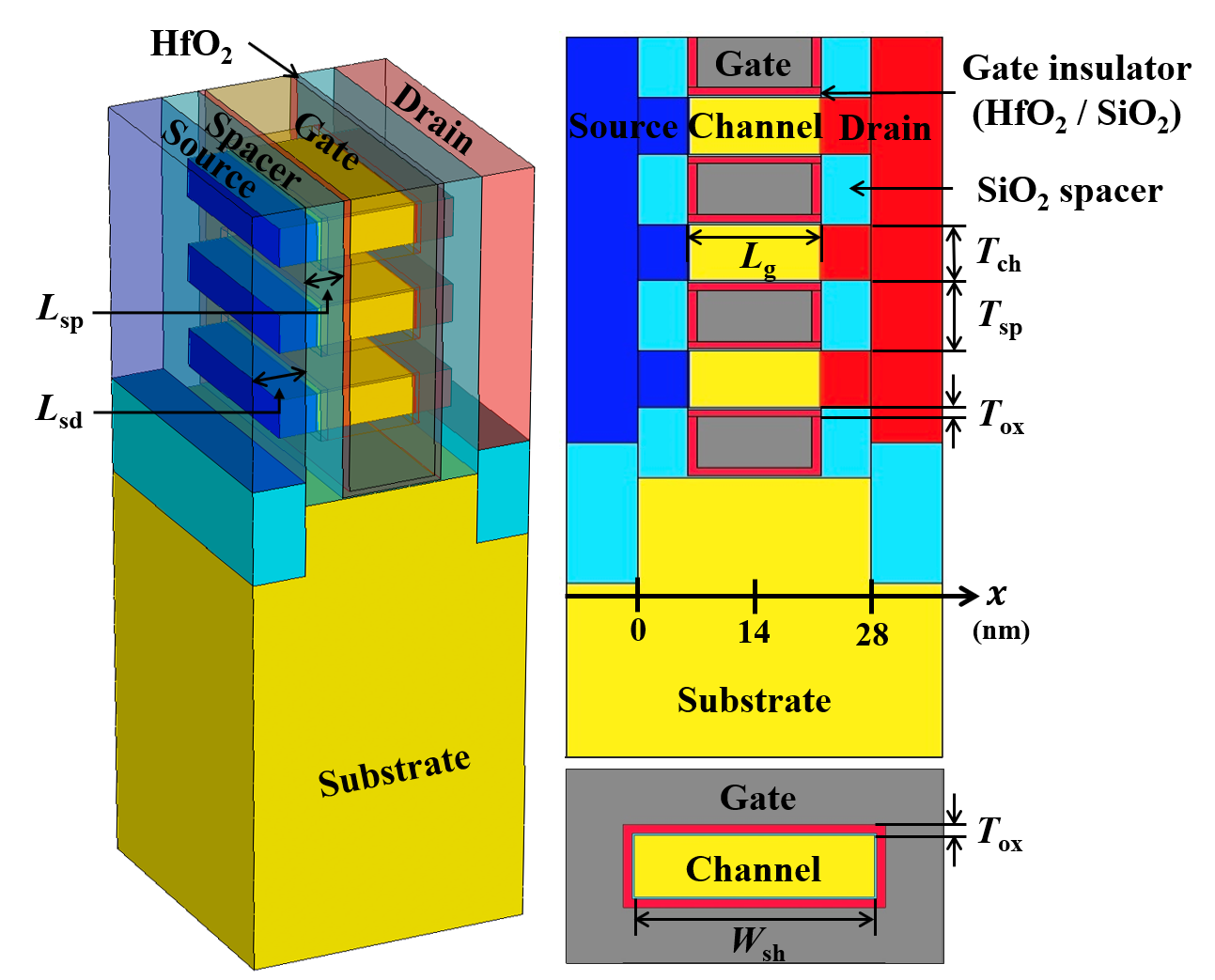##### Fig. 2. (a) The I$_{\mathrm{DS}}$-V$_{\mathrm{GS}}$ simulation curves fitted by the experimental data into implement the tunneling model. (b) The GB trap distribution extracted from the experimental data in.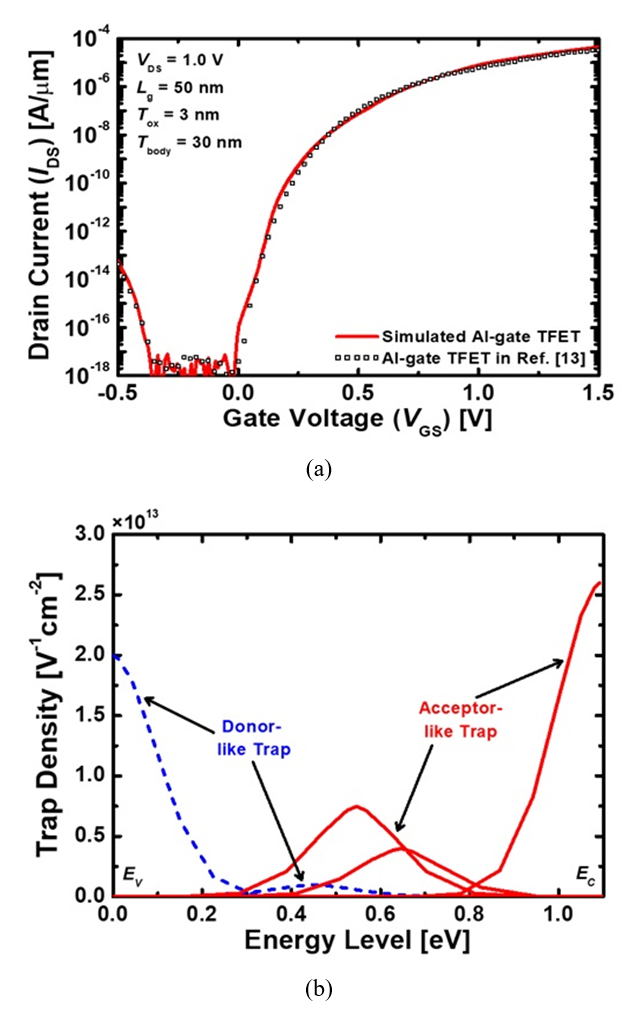##### Table 1. Parameters for the MNSTFET based on the poly-Si
 Parameter Value Gate length (Lg) 16 nm Spacer length (Lsp) 6 nm Extension source/drain length (Lsd) 8.5 nm Channel thickness (Tch) 8 nm Spacer thickness (Tsp) 10 nm Gate insulator (HfO2/SiO2) thickness (Tox) 1.1 / 0.3 nm Nanosheet width (Wsh) 30 nm Nanosheet total width (Wtotal) 76 nm p+ - Source doping concentration 1 × 1020 cm-3 n - Channel doping concentration 1 × 1016 cm-3 n+ - Drain doping concentration 1 × 1019 cm-3

## III. RESULTS AND DISCUSSION

Fig. 3(a)-(f) show the cross-sections of the MNSTFETs based on the poly-Si depending on the GB-locations. These devices have 3 nanosheets and they have 1 GB on each nanosheets except the case (f). Fig. 3(f) shows the device based on the single crystalline silicon without the GB. Fig. 4 is the transfer curves of the MNSTFETs for Fig. 3(a)-(f) cases, which are normalized with the W$_{\mathrm{total}}$. Table 2 summarizes the electrical characteristics of Fig. 4. Fig. 5(a) is the energy band diagrams of the MNSTFETs depending on the GB locations when the V$_{\mathrm{GS}}$ = 1 V. Fig. 5(b) is the energy band diagrams with enlarged the red boxes of Fig. 5(a). In Table 2, the I$_{\mathrm{on}}$ and the V$_{\mathrm{T}}$ in the case of x = 9 nm have the largest difference with 26.8\% and 5.0\%, respectively, compared with the case without the GB. The thicker the tunneling barrier, the more difficult it is for electrons to tunnel. The tunneling barrier for x = 9 nm is the thickest as shown as in Fig. 5(a) and it makes the I$_{\mathrm{on}}$ smaller and V$_{\mathrm{T}}$ larger. The tunneling barrier is thick because the sharp band peak is created due to electrons trapped in the GB as shown in Fig. 5(b). Therefore, the I$_{\mathrm{on}}$ is the lowest and the V$_{\mathrm{T}}$ is the largest. However, as shown in Fig. 5(b), when the GB location varies from 9 nm to 14, 19, and 24 nm in order, the sharp band peak gradually moves away from the tunneling region. This reduces the interference of the electron flow, so that the I$_{\mathrm{on}}$ increases and the V$_{\mathrm{T}}$ decreases, which is similar to the values in the case without the GB. Fig. 6(a) is the energy band diagrams of the MNSTFETs depending on the GB locations when the V$_{\mathrm{GS}}$ = 0 V. Fig. 6(b) is the energy band diagrams with enlarged the red boxes of Fig. 6(a). As shown in Table 2, the I$_{\mathrm{off}}$ and the average of subthreshold swing (SS$_{\mathrm{ave}}$) in the case of x = 24 nm have the largest difference of 49.2\% and 38.7\%, respectively, compared with the case without the GB. This is because the trap-assisted-tunneling (TAT) occurs when x = 24 nm. Fig. 6(a) shows that when x = 24 nm, it is the region where the TAT can occur. As shown in Fig. 6(b), when x = 24 nm, the energy band rises to the top due to the electrons trapped in the GB. The electrons are tunneled through these traps, and they result in high leakage current at an off-state. And the SS$_{\mathrm{ave}}$ is the largest value of 104.0 mV. Fig. 7(a) and (b) show the distribution of the electrons and holes trapped in the GB of the MNSTFETs when V$_{\mathrm{GS}}$ are 1.0 V and 0.0 V, respectively, depending on the GB locations. The energy band where the GB is located rises because the number of electrons trapped in the GB is larger than the number of holes. Thus, the energy band rises upwards, acting as if a negative voltage is applied.

We have compared the electrical characteristics of MNSTFETs and MNSMOSFETs. Fig. 8 shows the transfer curves of MNSMOSFETs based on the poly-Si depending on the GB-locations. The structure, the materials, and the GB locations of the MNSMOSFETs are the same as those of the MNSTFETs. Their doping concentrations in the source, channel, and drain regions are 1 ${\times}$ 10$^{19}$ cm$^{-3}$ (n-type), 1 ${\times}$ 10$^{17}$ cm$^{-3}$ (p-type), and 1 ${\times}$ 10$^{19}$ cm$^{-3}$ (n-type), respectively. In Fig. 9(a) and (b) compares the SS$_{\mathrm{min}}$ and the SS$_{\mathrm{ave}}$ of the MNSTFETs and the MNSMOSFETs depending on the GB locations. As shown in Fig. 9(a), the SS$_{\mathrm{min}}$’s for the MNSTFETs are smaller than those for the MNSMOSFETs. This is because the TFET uses tunneling mechanism and the MOSFET uses drift mechanism. Also, as shown in Fig. 9(b), the SS$_{\mathrm{ave}}$’s of MNSTFETs has a smaller effect depending on the GB locations than those of the MNSMOSFETs except for the case of x = 24 nm. In the case of MOSFET, the electrons trapped in the GB act the same as applying a negative voltage to the gate, interrupting channel formation . And it makes the SS$_{\mathrm{ave}}$ deviation larger depending on the GB locations. But, in the case of TFET, it is only affected when the GB is located in the TAT region. Therefore, if the GB is not located at 24 nm, the electrical characteristics of the MNSTFET depending on the GB locations are superior to those of the MNSMOSFET.

The poly-Si has the random distribution of the GB. Therefore, it is necessary to compare the characteristics of the MNSTFET and the MNSMOSFET by varying the GB location in each sheet. Fig. 10(a) and (b) show the histogram of the V$_{\mathrm{T}}$ and the SS$_{\mathrm{ave}}$ of the MNSTFETs and the MNSMOSFETs extracted from 125 (= 5 ${\times}$ 5 ${\times}$ 5) samples. The GB is located at one of the following locations on each sheet: 4, 9, 14, 19, and 24 nm. The total number of sheets of this device is 3, and each sheet has one GB. Fig. 10(c) shows a histogram of SS$_{\mathrm{ave}}$ of the MNSTFETs and the MNSMOSFETs extracted from 64 (= 4 ${\times}$ 4 ${\times}$ 4) samples except for the case of x = 24 nm in Fig. 10(b). The GB is located at one of the following locations on each sheet: 4, 9, 14, and 19 nm. The standard deviation (SD) is a value indicating how far the data are spread around the mean. The relative standard deviation (RSD) is the SD divided by the mean and multiplied by 100. The small RSD means that the SD is relatively small compared with the mean, so the

dispersion is small. In Fig. 10(a), the means of the extracted V$_{\mathrm{T}}$’s of the MNSTFETs and the MNSMOSFETs are 0.412 V and 0.206 V, respectively. The SDs are 3.90 mV and 8.31 mV, respectively. The RSDs are 0.95\% and 4.03\%, respectively. For the same reason as described for SS$_{\mathrm{ave}}$ in Fig. 9(b), the electrons trapped in the GB interfere with channel formation. So, the SD and RSD of the MNSTFET are smaller than those of the MNSMOSFET. In Fig. 10(b), the means of the extracted SS$_{\mathrm{ave}}$’s of the MNSTFETs and the MNSMOSFETs are 72.7 mV/dec and 70.5 mV/dec, respectively. The SDs are 7.08 mV/dec and 4.00 mV/dec, respectively. The RSDs are 9.75\% and 5.67\%, respectively. The SS$_{\mathrm{ave}}$’s of the MNSTFETs have a larger dispersion than those of the MNSMOSFETs. However, as shown in Fig. 10(c), except for the case of x = 24 nm, the means of the extracted SS$_{\mathrm{ave}}$’s of the MNSTFETs and the MNSMOSFETs are 67.2 mV/dec and 72.4 mV/dec, respectively. The SDs are 1.50 mV/dec and 4.16 mV/dec, respectively. The RSDs are 2.24\% and 5.74\%, respectively. If the GB is not located at 24 nm, the MNSTFET is better than the MNSMOSFET in terms of the I$_{\mathrm{off}}$, the V$_{\mathrm{T}}$, and the SS$_{\mathrm{ave}}$. Therefore, when fabricating the MNSTFET device, it is important to control the process so that the GB is not located in the drain region.

##### Table 2. The transfer characteristics of the MNSTFET depending on GB-locations
 x = Ion [μA/μm] Ioff[fA/μm] VT [V] SSmin [mV/dec] SSave[mV/dec] 4 nm 27.05 5.75 0.412 42.0 64.9 9 nm 23.40 7.38 0.425 43.1 68.9 14 nm 27.44 5.70 0.414 41.7 66.6 19 nm 28.57 5.96 0.407 40.2 69.5 24 nm 28.65 12.22 0.405 40.9 104.0 w/o GB 29.67 6.21 0.404 42.8 63.8
##### Fig. 3. (a) to (f) The cross-sections of the MNSTFET based on the poly-Si depending on GB-locations.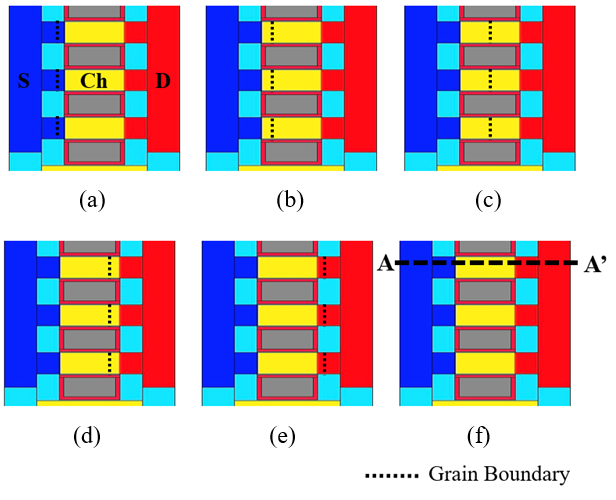##### Fig. 4. The transfer curves of the MNSTFET based on the poly-Si depending on GB-locations.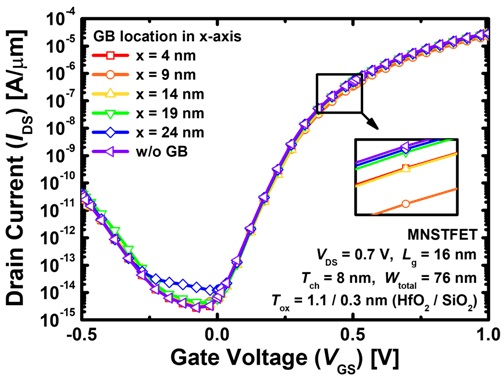##### Fig. 5. (a) The energy band diagram of the MNSTFET depending on GB-locations when V$_{\mathrm{GS}}$ is 1 V; (b) The energy band diagram with enlarged of the red boxes in Fig. 5(a). The energy bands are extracted at the 2 nm below the gate insulator along the A-A’ line.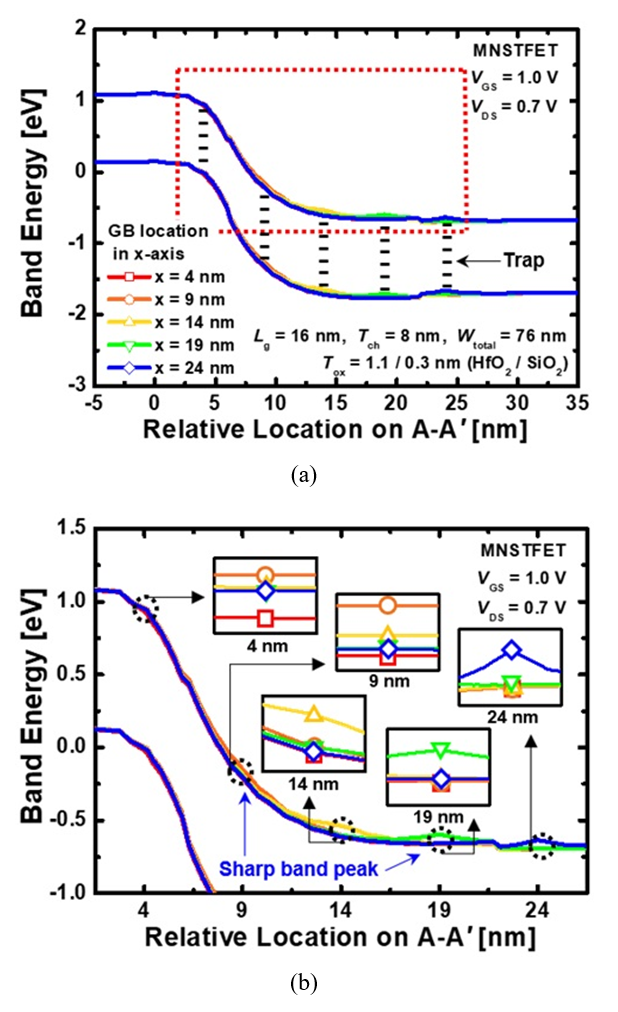##### Fig. 6. (a) The energy band diagram of the MNSTFET depending on GB-locations when V$_{\mathrm{GS}}$ is 0 V; (b) The energy band diagram with enlarged of the red boxes in Fig. 6(a).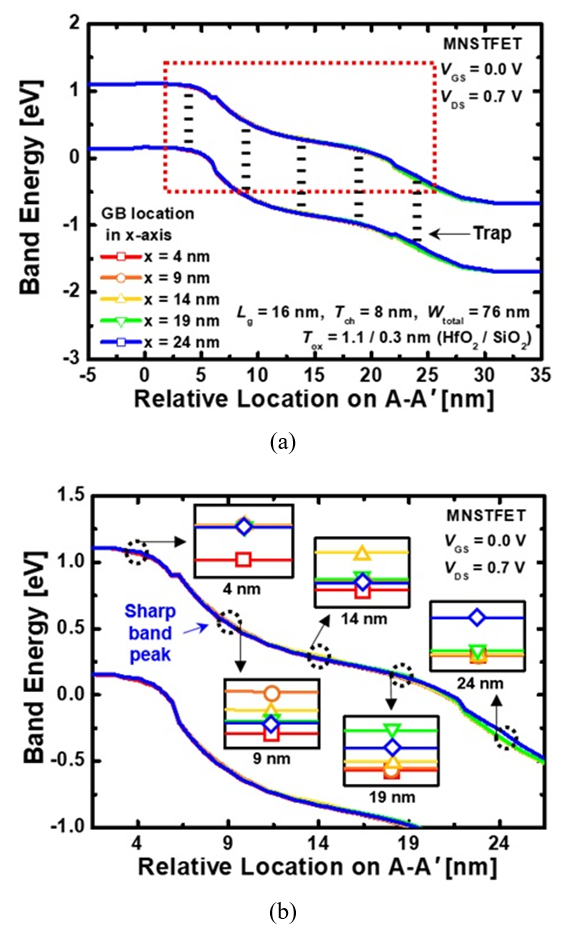##### Fig. 7. The distribution of electrons and holes trapped in the GB of the MNSTFET depending on GB-locations when V$_{\mathrm{GS}}$ is (a) 1 V; (b) 0 V, respectively. The electrons and holes trapped in GB are extracted at the 2 nm below the gate insulator along the A-A’ line.##### Fig. 8. The transfer curves of the MNSMOSFET based on the poly-Si depending on GB-locations.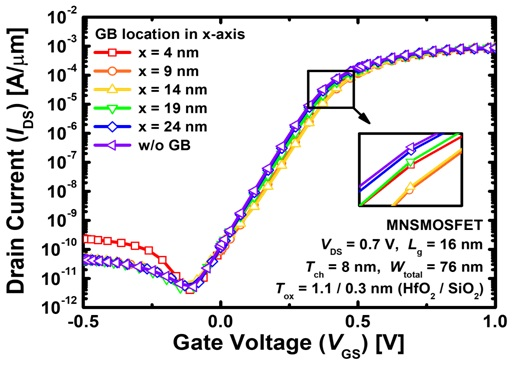##### Fig. 9. (a) SS$_{\mathrm{min}}$; (b) SS$_{\mathrm{ave}}$ of the MNSTFET and the MNSMOSFET based on the poly-Si depending on GB-locations.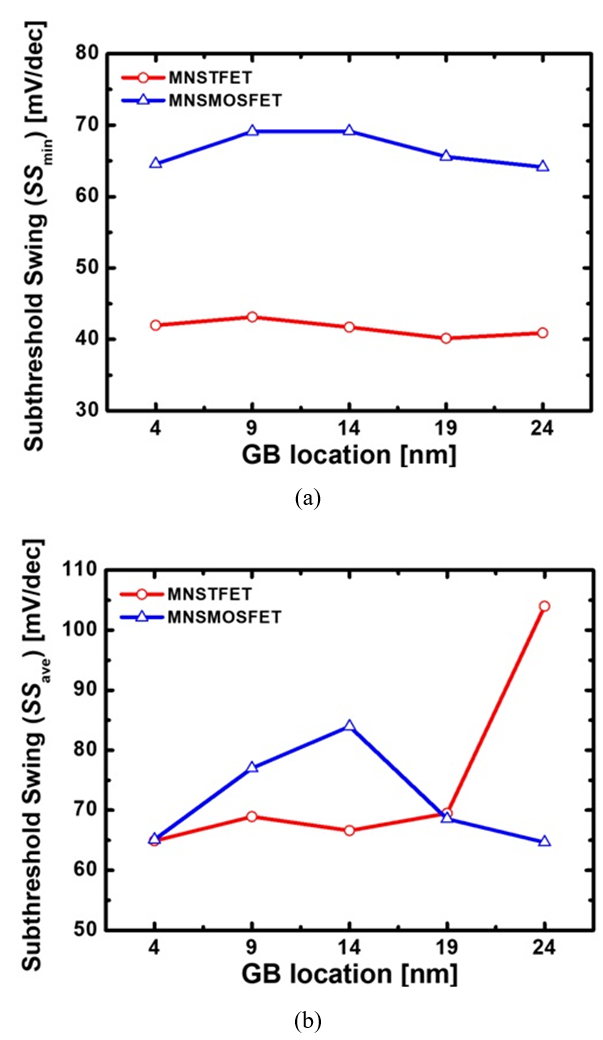##### Fig. 10. The histograms of (a) the V$_{\mathrm{T}}$’s; (b) the SS$_{\mathrm{ave}}$’s of the MNSTFETs and the MNSMOSFETs extracted from 125 (= 5 ${\times}$ 5 ${\times}$ 5) samples; (c) The histogram of SS$_{\mathrm{ave}}$’s of the MNSTFETs and the MNSMOSFETs extracted from 64 (= 4 ${\times}$ 4 ${\times}$ 4) samples except for the case when the GB is at 24 nm in Fig. 10(b).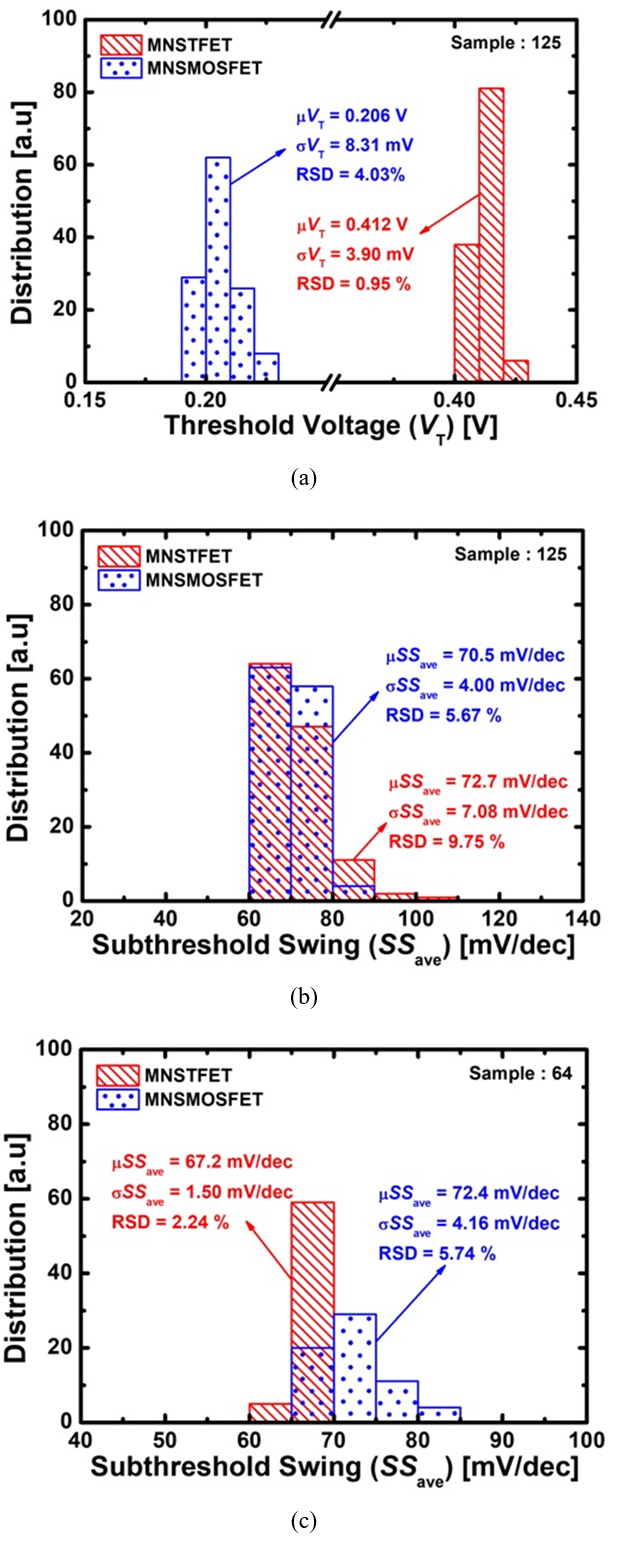## IV. CONCLUSIONS

This study showed the transfer performances depending on the GB-locations within a sheet in the MNSTFET based on the poly-Si. As a result of the analysis, the largest V$_{\mathrm{T}}$ was shown for the case of x = 9 nm, and the largest SS$_{\mathrm{ave}}$ was shown for the case of x = 24 nm due to the TAT. In addition, the electrical characteristics of 125 samples of the MNSTFETs were compared with the MNSMOSFETs. If the GB was not located at 24 nm, the MNSTFET was better than the MNSMOSFET in terms of the I$_{\mathrm{off}}$, the V$_{\mathrm{T}}$, and the SS$_{\mathrm{ave}}$. Consequently, this simulation showed that the MNSTFET has smaller effect on the GB location than the MNSMOSFET.

## ACKNOWLEDGMENTS

This work was supported by the National Research Foundation of Korea (NRF) grant funded by the Korea government (MSIT) (No. NRF-2020R1A2C1005087). This study was supported by the BK21 FOUR project funded by the Ministry of Education, Korea (4199990113966). This research was supported by Basic Science Research Program through the National Research Foundation of Korea (NRF) funded by the Ministry of Education (NRF-2021R1A6A3A13039927). This research was supported by National R&D Program through the National Research Foundation of Korea (NRF) funded by Ministry of Science and ICT (2021M3F3A2A03017764). This research was supported by National R&D Program through the National Research Foundation of Korea (NRF) funded by Ministry of Science and ICT (2022M3I7A1078936). This investigation was financially supported by Semiconductor Industry Collaborative Project between Kyungpook National University and Samsung Electronics Co. Ltd. The EDA tool was supported by the IC Design Education Center (IDEC), Korea.

## References

1
Khan, M. U., Arshad, A., Ume, S., Bukhari, R., Samer, A., and Tariq, F. “Nanoscale CMOS Scaling Trends, Challenges and Their Solutions.” ICAME21, International Conference on Advances in Mechanical Engineering, Pakistan, pp. 1-10, Aug. 2021.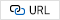2
Veeraraghavan, S., and Fossum, J. G. “Short-channel effects in SOI MOSFETs.” IEEE Transactions on Electron Devices, vol. 36, no. 3, pp. 522-528, Mar. 1989.3
Chandar, D. B., Vadthiya, N., Kumar, A., and Mishra, R. A. “Suppression of short channel effects (SCEs) by dual material gate vertical surrounding gate (DMGVSG) MOSFET: 3-D TCAD simulation.” Procedia Engineering, vol. 64, pp. 125-132, Nov. 2013.4
Bangsaruntip, S., Cohen, G. M., Majumdar, A., and Sleight, J. W. “Universality of short-channel effects in undoped-body silicon nanowire MOSFETs.” IEEE Electron Device Letters, vol. 31, no. 9, pp. 903-905, Sep. 2010.5
Choi, W. Y., Park, B. G., Lee, J. D., and Liu, T. J. K. “Tunneling field-effect transistors (TFETs) with subthreshold swing (SS) less than 60 mV/dec.” IEEE Electron Device Letters, vol. 28, no. 8, pp. 743-745, Aug. 2007.6
Zhang, Q., Zhao, W., and Seabaugh, A. “Low-subthreshold-swing tunnel transistors.” IEEE Electron Device Letters, vol. 27, no. 4, pp. 297-300, Apr. 2006.7
Appenzeller, J., Lin, Y. M., Knoch, J., Chen, Z., and Avouris, P. “Comparing carbon nanotube transistors-the ideal choice: a novel tunneling device design.” IEEE Transactions on Electron Devices, vol. 52, no. 12, pp. 2568-2576, Dec. 2005.8
Avci, U. E., Morris, D. H., and Young, I. A. “Tunnel field-effect transistors: Prospects and challenges.” IEEE Journal of the Electron Devices Society, vol. 3, no. 3, pp. 88-95, Apr. 2015.9
Ram, M. S., and Abdi, D. B. “Single grain boundary tunnel field effect transistors on recrystallized polycrystalline silicon: Proposal and investigation.” IEEE Electron Device Letters, vol. 35, no. 10, pp. 989-991, Oct. 2014.10
Ram, M. S., and Abdi, D. B. “Single grain boundary dopingless PNPN tunnel FET on recrystallized polysilicon: Proposal and theoretical analysis.” IEEE Journal of the Electron Devices Society, vol. 3, no. 3, pp. 291-296, May. 2015.11
Min, S. R., Lee, S. H., Park, J., Kim, G. U., Kang, G. E., Heo, J. H., Yoon, Y. J., Seo, J. H., Jang, J., Bae, J. H., and Kang, I. M. “Simulation of CMOS logic inverter based on vertically stacked polycrystalline silicon nanosheet gate-all-around MOSFET and its electrical characteristics.” Current Applied Physics, pp. 106-115, vol.43, Nov. 2022.12
Seon, Y., Chang, J., Yoo, C., and Jeon, J. “Device and circuit exploration of multi-nanosheet transistor for sub-3 nm technology node.” Electronics, vol. 10, no. 2, pp. 180, Jan. 2021.13
Cui, N., Liang, R., and Xu, J. “Heteromaterial gate tunnel field effect transistor with lateral energy band profile modulation.” Applied Physics Letters, vol. 98, no. 14, pp. 142105, Apr. 2011.14
Seo, J. H., Yoon, Y. J., Yu, E., Sun, W., Shin, H., Kang, I. M., Lee, J. H., and Cho, S. “Fabrication and characterization of a thin-body poly-Si 1T DRAM with charge-trap effect.” IEEE Electron Device Letters, vol. 40, no. 4, pp. 566-569, Apr. 2019.15
Sentaurus User's Manual, Version L-2016.03, Synopsys, Mountain View, CA, USA, Mar. 2016.Ga Eon Kang received a B.Sc. degree in electronic engineering from the School of Electronics Engi-neering (SEE), Kyungpook National University (KNU), Daegu, South Korea, in 2022, where she is currently pursuing a M.S. degree in electronic and electrical engineering. Her research interests include the design, fabrication, and characterization of GaN devices and tunneling FETs.

Sang Ho Lee received a B.Sc. degree in electronics engineering from the School of Electronics Engineering (SEE), Kyungpook National University (KNU), Daegu, South Korea, in 2019, where he is currently pursuing a Ph.D. in electronic and electrical engineering with the SEE. His research interests include the design, fabrication, and characterization of gate-all-around logic devices and capacitor-less 1T-DRAM transistors.

Jin Park received a B.Sc. degree in electronic engineering from the School of Electronics Engineering (SEE), Kyungpook National Univer-sity (KNU), Daegu, South Korea, in 2020, and a M.Sc. degree from the school of electronic and electrical Engineering (SEE), Kyungpook National University (KNU), where she is currently pursuing a Ph.D. in electronic and electrical engineering. Her research interests include the design, fabrication, and characterization of gate-all-around logic devices and capacitor-less 1T-DRAM transistors.

So Ra Min received a B.Sc. degree in Electronic Engineering from the School of Electronics Engineering, Yeungnam University (YU), Gyeong-san, North Gyeongsang, South Korea, in 2020, where she is currently pursuing a M.Sc. degree in school of Electronic and Electrical Engineering. Her research interests include the design, fabrication, and characterization of GaN devices and capacitor-less 1T-DRAM transistors.

Geon Uk Kim received a B.Sc. degree in electronic engineering from the School of Electronics Engi-neering (SEE), Kyungpook National University (KNU), Daegu, South Korea, in 2021, where he is currently pursuing an M.S. degree in electronic and electrical engineering. His research interests include the design, fabrication, and characterization of GaN devices and capacitor-less 1T-DRAM transistors.

Jun Hyeok Heo received a B.Sc. degree in electronic engineering from the School of Electronics and Information Engineering, Korea University (KU) Sejong Campus, Sejong-si, South Korea, in 2021, where he is currently pursuing an M.S. degree in electronic and electrical engineering. His research interests include the design, fabrication, and characterization of vertical GaN power devices.

Jae Won Jang received the B.S. and M.S degrees in electrical engineering from Korea University, Seoul, Korea in 2006 and 2008, respectively. In 2013, Jaewon Jang received Ph.D degrees in electrical engineering and computer sciences from University of California at Berkeley, CA, USA. From 2013 to 2014, he was a post doctorial researcher, and working for developing of high-performance metal oxide transistors by printing technology. From 2015 to 2016, he was a researcher and working for developing of high performance organic thin film transistor in Samsung Advanced Institute and Technology, Suwon, Korea. Since 2016, he has been with Kyungpook National University, Daegu, Korea, where he is currently an assistant professor with the School of Electronics Engineering.

Jin-Hyuk Bae received a B.S. degree in Electronics and Electrical Engineering from Kyungpook Na-tional University, Daegu, Korea in 2004, and M.S. and Ph.D. degrees in Electrical Engineering from the Seoul National University, Seoul, Korea in 2006 and 2010, respectively. For the period from 2010 to 2012, he worked as a post-doctoral research fellow with Ecole Nationale Superiere des Mines de Saint-Etienne, Gardanne, France. In 2012, he joined the faculty in the School of Electronics Engineering, Kyungpook National University, Korea, where he is currently an Associate Professor. His research interests include interfacial engineering and physics of organic based and metal-oxide-based electronic devices and their sensor applications.

Sin-Hyung Lee received his B.S. and Ph.D. degrees in electrical engineering from Seoul National University, Korea in 2013 and 2019, respectively. He is currently an assistant professor in the School of Electronics Engineering at Kyung-pook National University in Republic of Korea. His research covers the neuromorphic electronics, artificial synapse, memristors, and organic electronics.

In Man Kang received the B.S. degree in electronic and electrical engineering from School of Elec-tronics and Electrical Engineering, Kyungpook National University (KNU), Daegu, Korea, in 2001, and the Ph.D. degree in electrical engin-eering from School of Electrical Engineering and Computer Science (EECS), Seoul National University (SNU), Seoul, Korea, in 2007. He worked as a teaching assistant for semiconductor process education from 2001 to 2006 at Inter-university Semiconductor Research Center (ISRC) in SNU. From 2007 to 2010, he worked as a senior engineer at Design Technology Team of Samsung Electronics Company. In 2010, he joined KNU as a full-time lecturer of the School of Electronics Engineering (SEE). Now, he is currently working as an associate professor. His current research interests include CMOS RF modeling, silicon nanowire devices, tunneling transistor, low-power nano CMOS, and III-V compound semiconductors. He is a member of IEEE EDS.